# Cracking The Rooted Tree - Discrete Mathematics Code | GlobalEduCore

## ROOTED TREES

Many of the applications of graph theory, particularly in computer science, use a certain kind of tree, called a rooted tree. These trees are used as models for the structures of file
directories. Some of the other important uses of rooted trees include the representation and
sorting of data, the representation of algebraic expressions etc.
A tree in which one vertex (called the root) is distinguished from all the other vertices is known as rooted tree.
Trees without any root are called free trees or simply trees. A
vertex of degree one which is not a root is called a leaf or external node and all the vertices
including the roots) that are not leaves are called interior nodes. For instance in Fig.7.1 below a vertex v is a root for all the trees shown.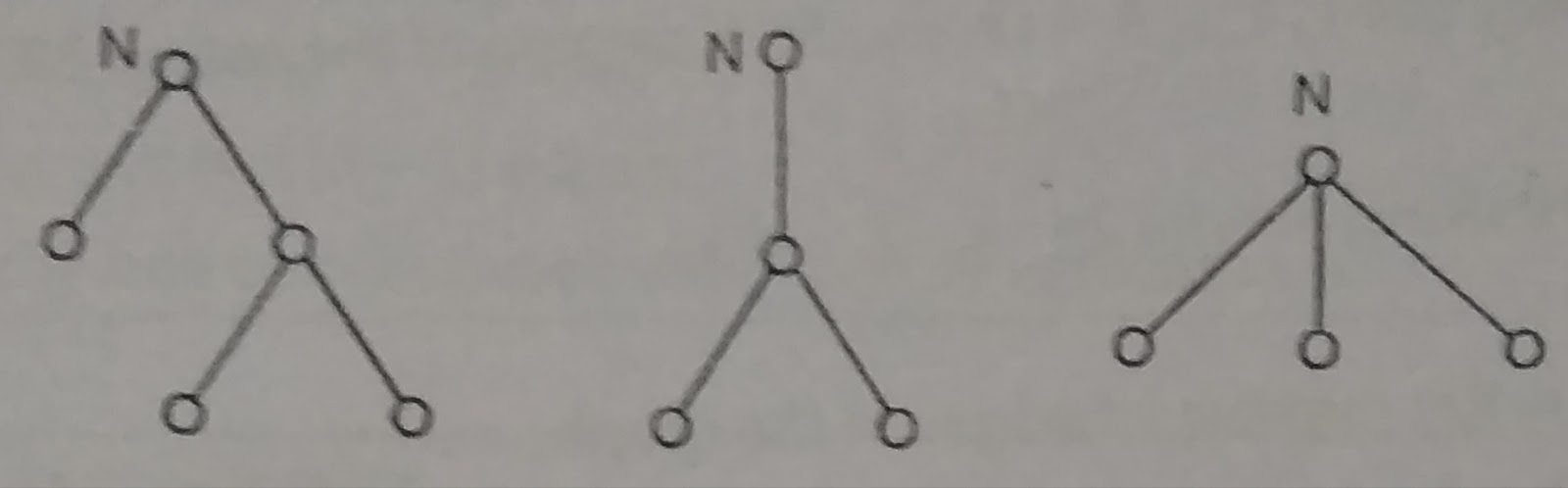Fig 7.1

The other example of rooted tree is sorting of mail according to the zip code.
Consider the Fig. 7.1The point N from where all the mail goes out is distinguished from the rest of the vertices. Hence N can be considered as the root of the tree.
A rooted tree is often used to specify hierarchical relationships.
A example of such a tree, which is an organization chart of a corporation is given in the following Fig

Directed Tree
A directed tree (a tree with directions) is called a rooted tree if there is exactly one vertex
whose incoming degree is zero and ail other vertices have incoming degree one The vertex
with incoming degree 0 is known as the root of the tree In Fig 7.15 the vertex a is the root
of the directed tree.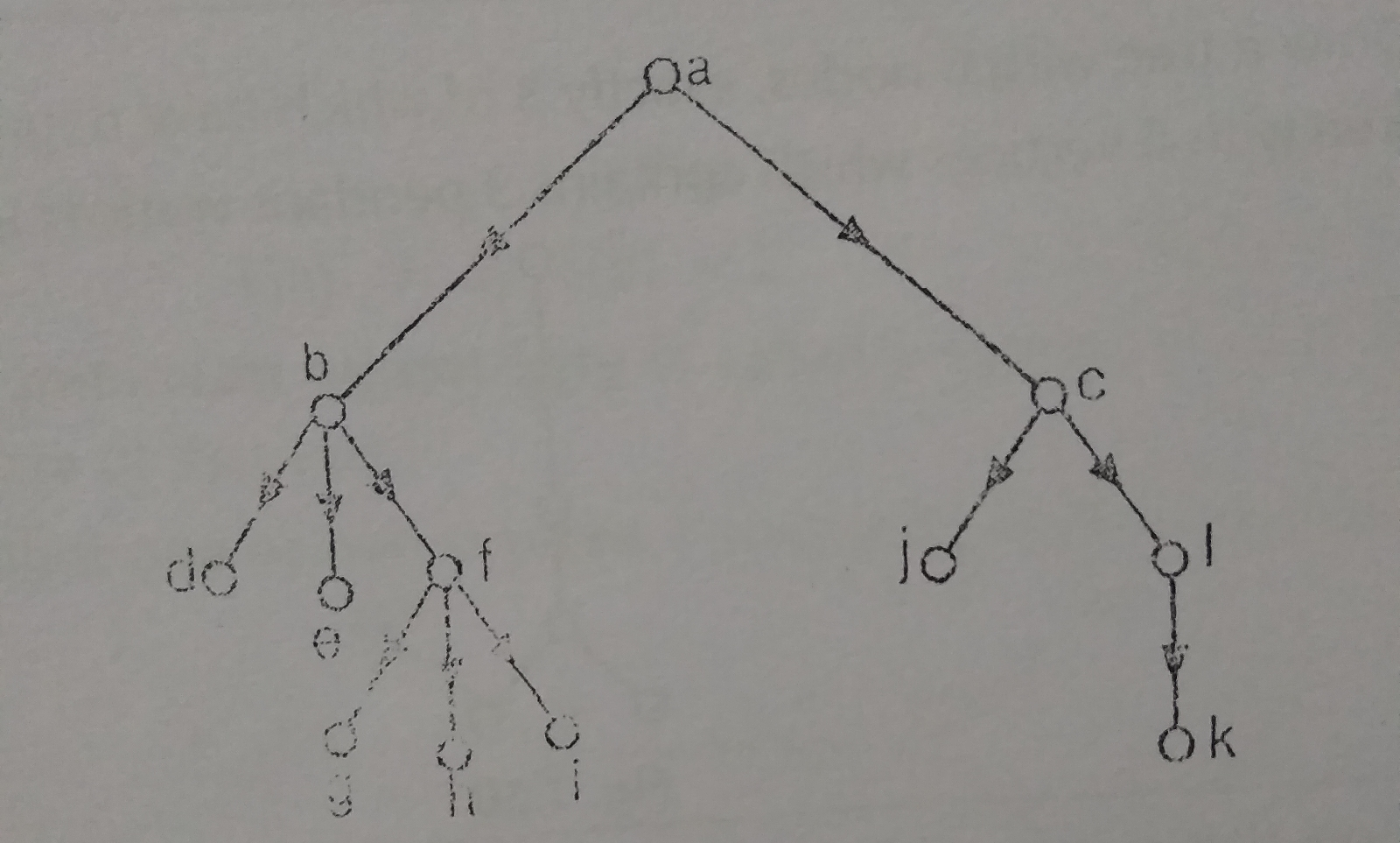Fig 7.15

Fig. 7 15
In a directed rooted tree, a vertex whose outgoing degree is 0 is called a leaf and a vertex
whose outgoing degree is non zero is called a branch node or an internal node In Fig. 7.15
the vertices b, c and f are branch nodes and the vortices d, e, g are leaves.
Now we are in position to defne the level of a vertex in a rooted tree.
A vertex x ina rooted tree is said to be at level n if there
is a path of length n from the root to the vertex x. The
height of the tree is the maximum of the levels-of-its
vertices. Height of the tree is also called the depth of the
tree. In the following Fig. 7.16, b is at level 1 and g is at
level 3 The height of the tree IS 3.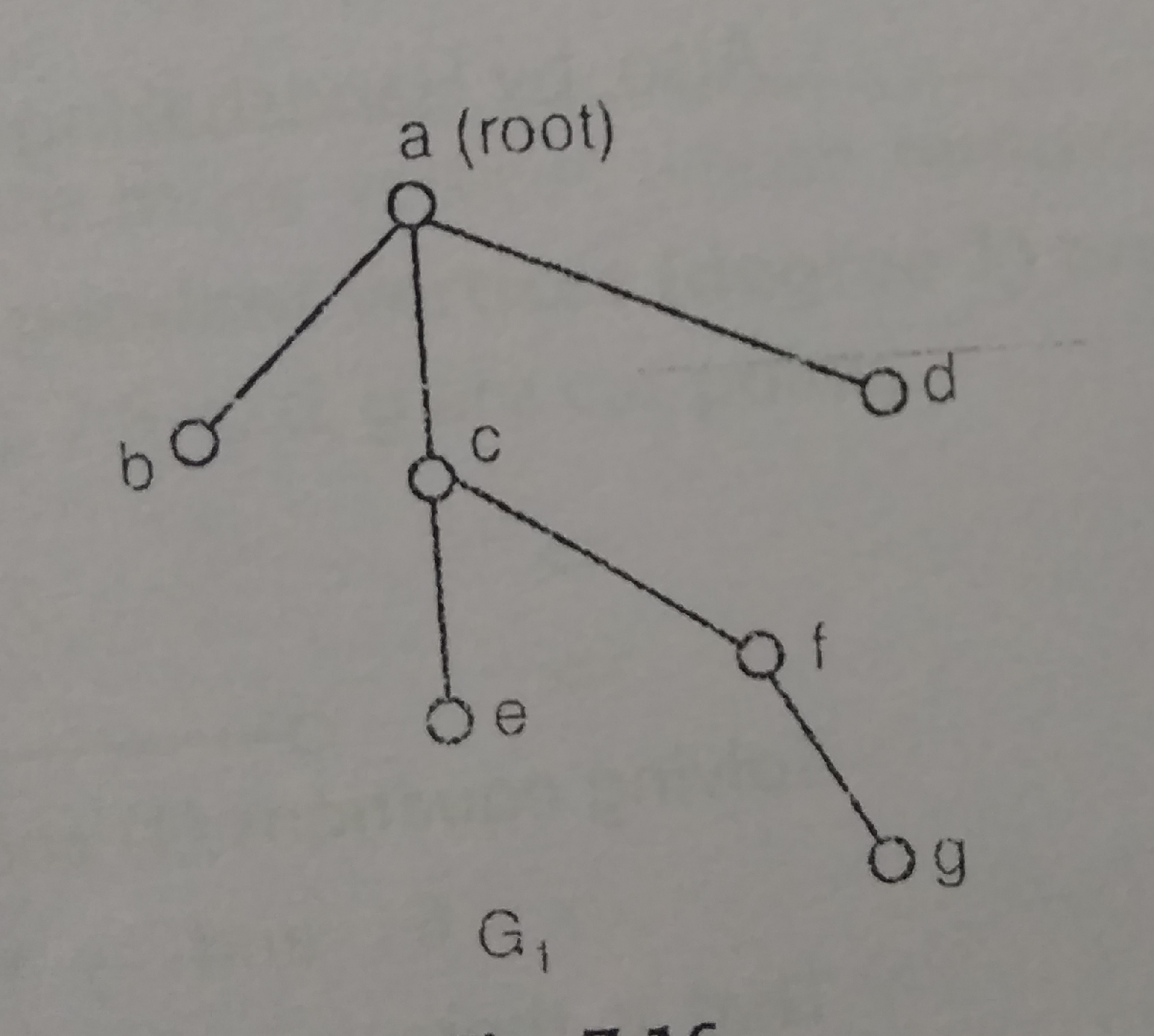fig.7.16

In a rooted tree, if the level of a vertex y in greater than the level of the vertex x, then we say
y is below x. If y is below x and there is an edge from x to y, then we say y is on of x.
Also, x is said to be the father of y. Two vertices are said to be brothers if they are sons of
the same vertex If P = (X, V V2 - Vn- y) is a path from « to y, then y is called a
descendant of x. Also, x is said to be an ancestor of y. These terms clearly indicate that
family trees are indeed rooted trees. To understand these terms, consider rooted tree G, in e verter c Hence e is the son of cor cis the father of e. Also
Fig. 716. The vertex e is below
e and f are the sons of c. Therefore the vertices e and fare brothers. Also, the vertices c and g are connected by the sequence of edges and is below c Therefore, g is a descendant of c or we can say cis an ancestor of g.

The degree of a tree is the maximum degree of the nodes in the tree In Fig 7.16, the degree of the tree is 3

A forest is a set of disjoint trees If we remove the root of a tree, we get a forest i.e. set of disjoint trees.

In another words, if the root and corresponding cdges connecting the nodes (sons) to the root are deleted from a tree, we obtain a set of disjoint trees. This set of disjoint trees is
called a forest
"We present now the definitions of subtree and m ary tree in a rotated tree" in a rooted tree T, a vertex x, together with all its descendants, is calied the subtree of T
rooted at x.
Following Fig 7.17 shows the rooted tree T, and its subtree T' rooted at b.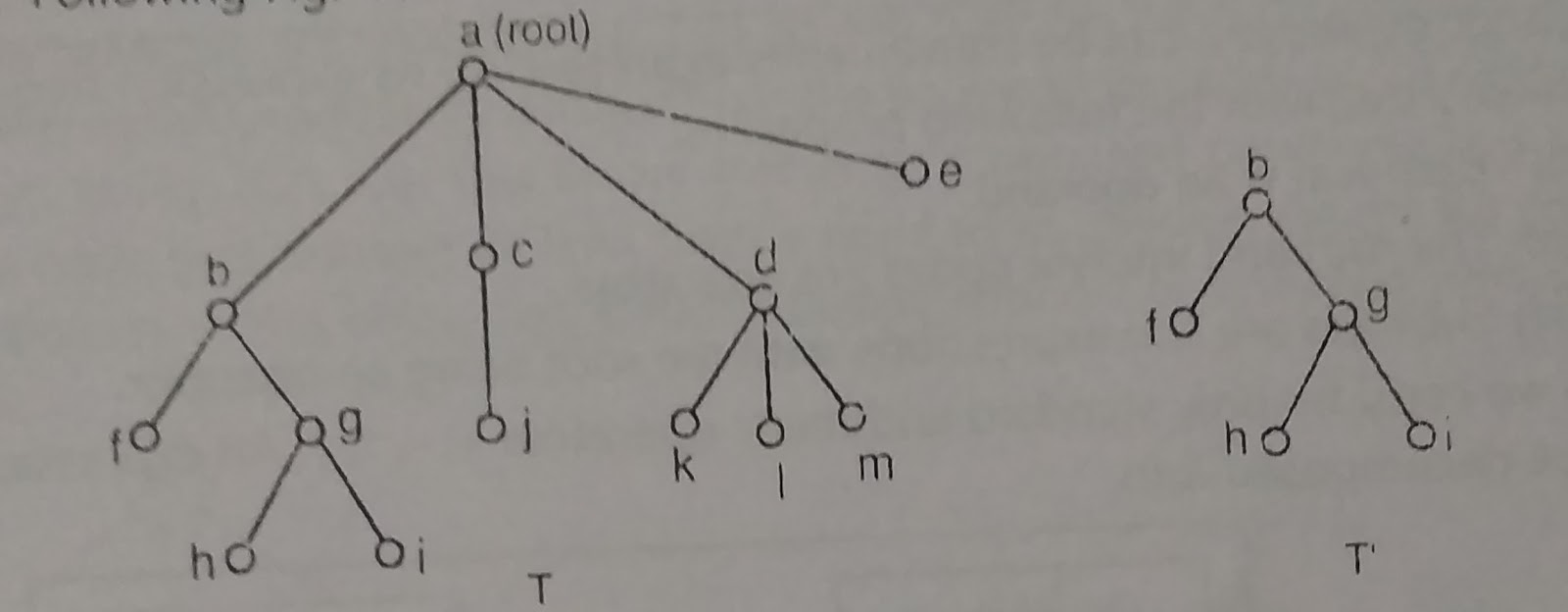Fig 7.17

A rooted tree in which every interior node las atmost m sons is called an m-ary tree.

An m-ary tree is said to be regular m-ary tree or full m-ary tree if every branch node has exactly m sons. For example, in the following Fig. 718 G, is a 2 - ary tree or binary tree but it is not a regular binary tree. G, is a regular 3 - ary tree or a ternary tree.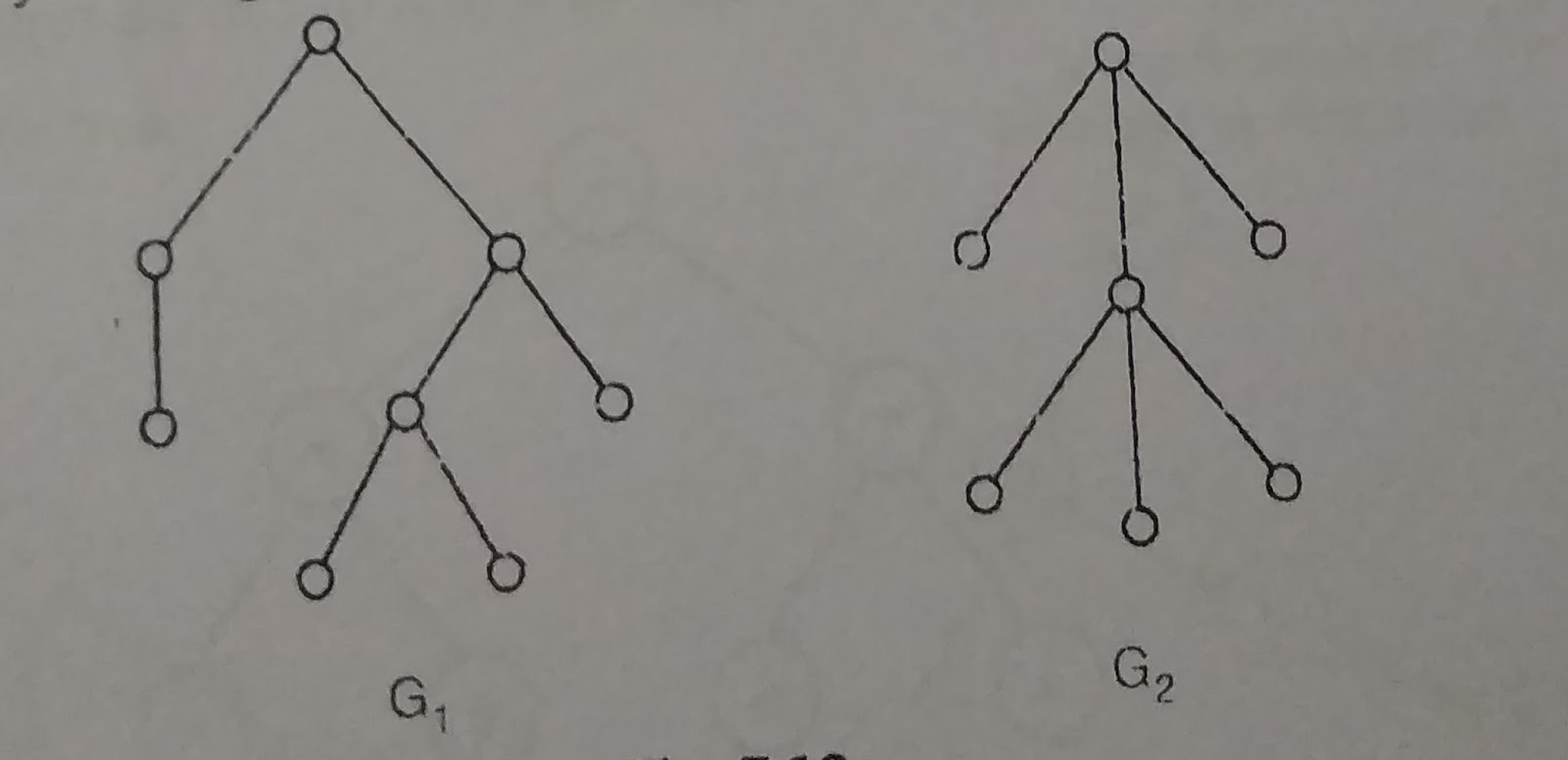Fig 7.18
In a regular m-ary tree, the relation between the interior nodes and the total number of nodes is given by following theorem.

Theorem
A regular m-ary tree with i interior nodes has (mi + 1) nodes at all.
Proof:
Suppose the given regular m-ary tree has n number of vertices, out of which there are i interior vertices.

Clearly, the number of leaves or sons in the tree is t = (n-i).
Since the given graph is a regular m-ary tree hence each of the i interior nodes has m sons
and thus the regular m-ary tree will have total (mi) sons.
But root is not a son.
Therefore the given tree has a total of (mi + 1) number of vertice(s).
Hence n = mi + 1

[blogger]

## Contact Form

Name

Email *

Message *

Javascript DisablePlease Enable Javascript To See All Widget